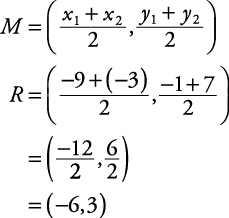## Midpoint Formula

Numerically, the midpoint of a segment can be considered to be the average of its endpoints. This concept should help in remembering a formula for finding the midpoint of a segment, given the coordinates of its endpoints. Recall that the average of two numbers is found by dividing their sum by two.##### Example 1

In Figure 1, R is the midpoint between Q(–9,–1) and T(–3,7). Find its coordinates.

By the midpoint formula,Back to Top
A18ACD436D5A3997E3DA2573E3FD792A